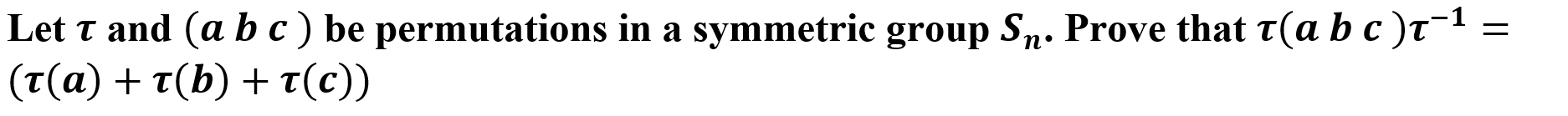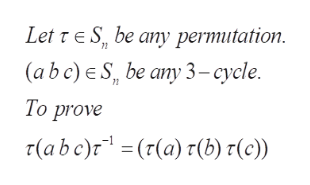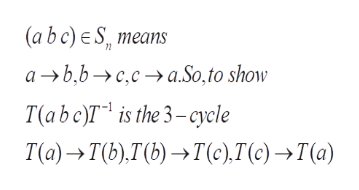Let T and (a bc) be permutations in a symmetric group Sn. Prove that T(a b c)t1(τ(α) +τ(b) + τ(c)

Question

I need help solving/ understanding attached for abstarct algebra dealing with permutations

Thankshelp_outlineImage TranscriptioncloseLet T and (a bc) be permutations in a symmetric group Sn. Prove that T(a b c)t1 (τ(α) +τ(b) + τ(c) fullscreen
Step 1

To prove the property of conjugation of a 3-cycle in the Symmetric group

Step 2

Problem statement (note the correction , there is no + sign; it doesnt make any sense). For convenience, we will denote tau as T, in what follows.help_outlineImage TranscriptioncloseLet r E S, be any permutation (a b c) e S be any 3-cycle. 72 То prove 7(abe)r(r(a) r(b) 1(c)) fullscreen
Step 3help_outlineImage Transcriptionclose(a bc) e S, means а >ь,ь >сса.So,to show T(аb c)T* is the 3- сyele T(а) > T),Г) —т(), T() —Т(а) fullscreen

Want to see the full answer?

See Solution

Want to see this answer and more?

Our solutions are written by experts, many with advanced degrees, and available 24/7

See Solution
Tagged in

Math